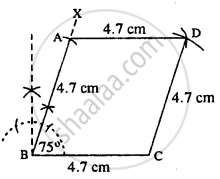# Construct a Rhombus Abcd, If ; Bc = 4.7 Cm and ∠B = 75°. - Mathematics

Sum

Construct a rhombus ABCD, if ;

BC = 4.7 cm and ∠B = 75°.

#### SolutionSteps :

1. Draw BC = 4.7 cm.
2. At B, draw ∠XBC = 75°
3. Cut BA = 4.7 cm.
4. From A and C, draw arcs of radii 4.7 cm each which intersect at D.